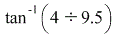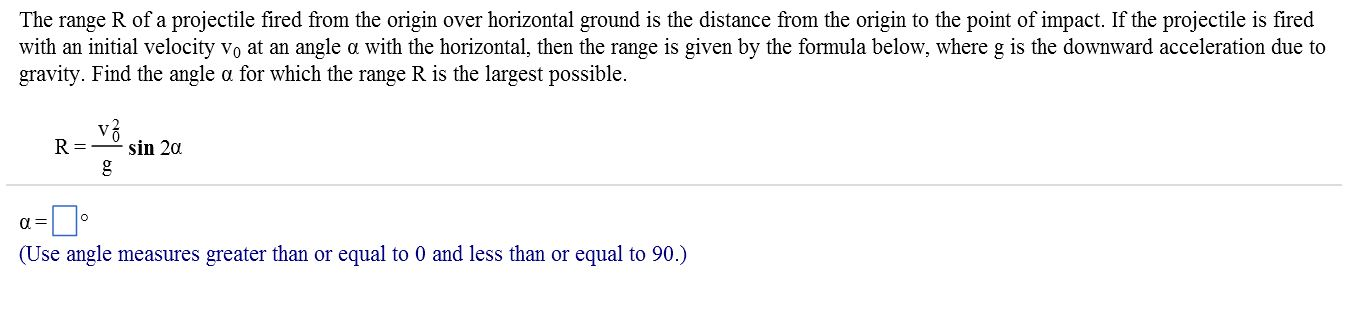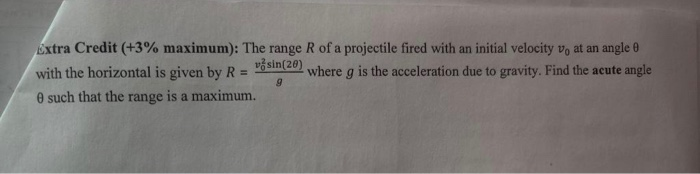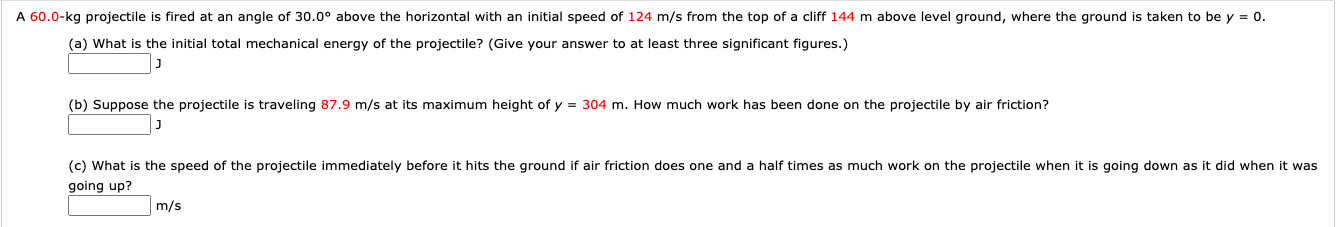Question

# A projectile is fired in such a way that its horizontal range is equal to 9.5...

A projectile is fired in such a way that its horizontal range is equal to 9.5 times its maximum height. What is the angle of projection?

Horizontal Range = v2 sin2θ/g

Height = v2 sin2θ/2g

According to the question

v2 sin2θ/g= 9.5 x v2 sin2θ/2g

=>2v2 sinθcosθ/g= 9.5 x v2 sin2θ/2g

=> 4/9.5=sinθ/cosθ [On Simpplification]

=>tanθ=4/9.5=> θ==22.830

Hence the angle of Projection is 22.830 with respect to the horizontal.

#### Earn Coins

Coins can be redeemed for fabulous gifts.

Similar Homework Help Questions
• ### a projectile is fired in such a way that its horizontal range is equal to three times its...

a projectile is fired in such a way that its horizontal range is equal to three times its maximum hight. what is the angle of projection?

• ### A projectile is fired with an initial speed of 10 m/s. Find theangle of projection...

A projectile is fired with an initial speed of 10 m/s. Find the angle of projection such that the maximum height of the projectile is equal to 0.9 times its horizontal range.

• ### the horizontal range of a projectile is 4 root 3 times of its maximum height

the horizontal range of a projectile is 4 root 3 times of its maximum height. calculate the angle of projection.

• ### prove that the maximum horizontal range is 4 times the maximum height attained by a projectile which is fired long the required oblique direction

prove that the maximum horizontal range is 4 times the maximum height attained by a projectile which is fired long the required oblique direction

• ### please show worked problem; The range R of a projectile fired from the origin over horizontal...please show worked problem; The range R of a projectile fired from the origin over horizontal ground is the distance from the origin to the point of impact. If the projectile is fired with an initial velocity v0 at an angle a with the horizontal, then the range is given by the formula below, where g is the downward acceleration due to gravity. Find the angle a for which the range R is the largest possible. R = v^2/g sin...

• ### A projectile fired from a gun has initial horizontal and vertical components of velocity equal to...

A projectile fired from a gun has initial horizontal and vertical components of velocity equal to 30 m/s and 40 m/s, respectively. a. determine the initial speed of the projectile b. at what angle is the projectile fired (measured with respect to the horizontal)? c. approximately how long does it take the projectile to reach the highest point in its trajectory?

• ### A projectile is fired from the ground (you can assume the initial height is the same...

A projectile is fired from the ground (you can assume the initial height is the same as the ground) in a field so there are no obstacles in its way. It is fired at an angle of 24° with respect to the horizontal and with an initial speed of 25 m/s. Air resistance is negligible in this situation. Call up the positive y-direction, and toward the wall the positive x-direction. What is the earliest time when the height of the...

• ### A projectile is fired from the ground (you can assume the initial height is the same...

A projectile is fired from the ground (you can assume the initial height is the same as the ground) in a field so there are no obstacles in its way. It is fired at an angle 11° with respect to the horizontal and with an initial speed of 46 m/s. Air resistance is negligible in this situation. Call up the positive y direction, and toward the wall the positive x direction. What is the earliest time when the height of...

• ### Extra Credit (+3% maximum): The range R of a projectile fired with an initial velocity vo...Extra Credit (+3% maximum): The range R of a projectile fired with an initial velocity vo at an angle with the horizontal is given by R = ama where g is the acceleration due to gravity. Find the acute angle such that the range is a maximum.

• ### A 60.0-kg projectile is fired at an angle of 30.0° above the horizontal with an initial...A 60.0-kg projectile is fired at an angle of 30.0° above the horizontal with an initial speed of 124 m/s from the top of a cliff 144 m above level ground, where the ground is taken to be y = 0. (a) What is the initial total mechanical energy of the projectile? (Give your answer to at least three significant figures.) (b) Suppose the projectile is traveling 87.9 m/s at its maximum height of y = 304 m. How much...